# OpenCV-Python霍夫线变换

• 我们将理解霍夫变换的概念。
• 我们将看到如何使用它来检测图像中的线条。
• 我们将了解以下函数： cv.HoughLines（）cv.HoughLinesP（）

## 霍夫变换理论

y = mx+c

\rho = x \cos \theta + y \sin\theta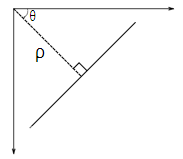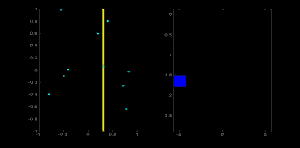## OpenCV 中的霍夫变换

import cv2 as cv
import numpy as np
gray = cv.cvtColor(img,cv.COLOR_BGR2GRAY)
edges = cv.Canny(gray,50,150,apertureSize = 3)
lines = cv.HoughLines(edges,1,np.pi/180,200)
for line in lines:
rho,theta = line
a = np.cos(theta)
b = np.sin(theta)
x0 = a*rho
y0 = b*rho
x1 = int(x0 + 1000*(-b))
y1 = int(y0 + 1000*(a))
x2 = int(x0 - 1000*(-b))
y2 = int(y0 - 1000*(a))
cv.line(img,(x1,y1),(x2,y2),(0,0,255),2)
cv.imwrite('houghlines3.jpg',img)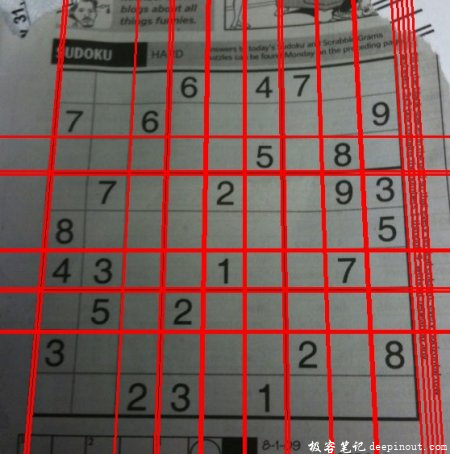## 概率 Hough 变换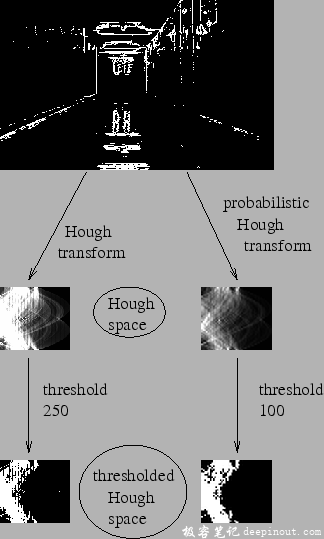OpenCV 实现基于使用 Matas，J。和 Galambos，C。和 Kittler，J.V。的渐进概率 Hough 变换的线的鲁棒检测。使用的函数是 cv.HoughLinesP（） 。它比之前介绍的函数多出来两个参数：

• minLineLength – 最小线长。比这个值小的线条会被丢弃。
• maxLineGap – 允许线段之间的最大间隙，以便将(在同一条直线上的)线段视为同一条。

import cv2 as cv
import numpy as np
gray = cv.cvtColor(img,cv.COLOR_BGR2GRAY)
edges = cv.Canny(gray,50,150,apertureSize = 3)
lines = cv.HoughLinesP(edges,1,np.pi/180,100,minLineLength=100,maxLineGap=10)
for line in lines:
x1,y1,x2,y2 = line
cv.line(img,(x1,y1),(x2,y2),(0,255,0),2)
cv.imwrite('houghlines5.jpg',img)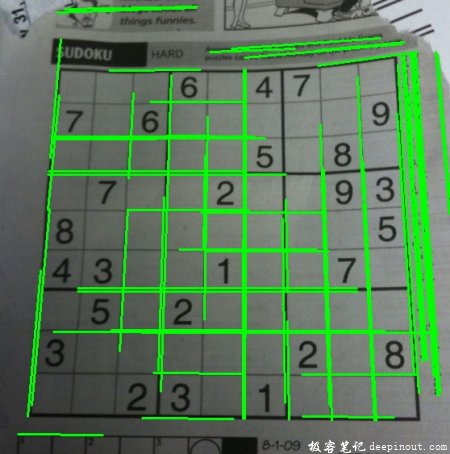• 回顶